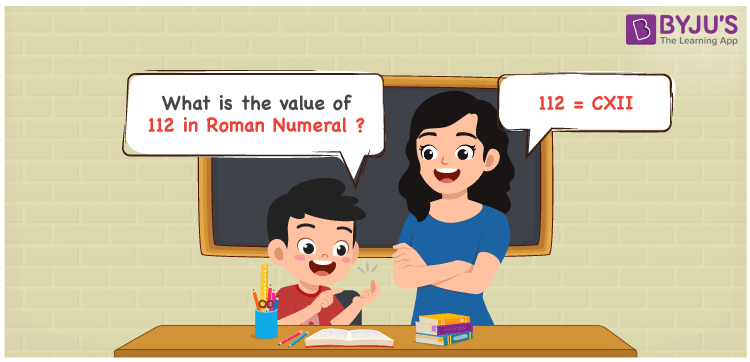# 112 in Roman Numerals

112 in Roman Numerals is CXII. Any Roman numeral uses only the 7 primary symbols which are I, V, X, L, C, D, and M. Here C represents 100, X represents 10 and I represents 1. 112 can be obtained by adding the 3 primary symbols C, X and I. For understanding the rules on Roman Numerals, you can refer to the link Rules to write Roman Numerals. The details on how to write 112 is given in the next section of this article.

 Number Roman Numeral 112 CXII

## How to Write 112 in Roman Numerals?To convert 112 in Roman Numerals, we need to represent 112 as the sum of the fundamental symbols. 112 is nothing but 100 + 10 + 2, both 100 and 10 have the primary symbols, But 2 does not have. So 2 can be represented as twice I.

112 = 100 + 10 + 2

112 = 100 + 10 + 1 + 1

112 = C + X + I + I

112 = CXII

## Video Lesson on Roman Numerals## Frequently Asked Questions on 112 in Roman Numerals

Q1

### How to write the number 112 in Roman Numerals?

112 in Roman Numerals is written as CXII.
Q2

### Does CXII represent Roman Numerals?

Yes. CXII represents Roman Numerals and it is the number 112
Q3

### How to read the Roman Numeral CXII in English?

The Roman Numeral CXII represents 112 and it is read as One Hundred and Twelve in Roman Numerals is CX.